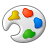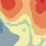# Residual-kriging

1087
3
03-08-2012 12:21 AMby
New Contributor
Hi,

I want to do a resudal-kriging over an area in Arcgis between point values (measured precipitation at different points). It want to do that by first customize a plan of the precipitation by for example making regression on the following equation: p = a*x + b*y + c (where p is the precipitation and x and y are the coordinates) and then kriging the residuals.

Is the method I describe the same as "Universal Kriging" in ArcMap, with first order of trend removal?

Kindly regards
Peter
Tags (3)
3 RepliesbyEsri Regular Contributor
Yes, the model you just described is Universal kriging with first-order trend removal, as implemented in ArcGIS (other implementations allow covariates other than just the x,y coordinates).  In the Kriging tool in Spatial Analyst, a global first- or second-order trend is fit to the x,y coordinates.  In Geostatistical Analyst, you can do local trend removal (ie, the coefficients of the x,y coordinates in the model will change across the surface).by
New Contributor
What is the difference between constant trend removal, and first order of trend removal?byEsri Regular Contributor
Constant trend removal either adds or subtracts a constant value to the whole surface (if it is done globally).  This value is the same everywhere in the surface.  A first-order trend removal will use the X,Y coordinates as predictors in a first-order linear model.

Models:
Constant: z = Intercept + Error
First-order: z = Intercept + aX + bY + Error

...where z is the variable you are interpolating, and a,b are coefficients that are calculated using ordinary least-squares.  Kriging is then performed on the Error term.  When the trend removal is done globally (as in the Kriging tool in Spatial Analyst), the values of [Intercept, a, b] will be the same across the entire surface.  When you do a local trend removal (only in Geostatistical Analyst), the values of [Intercept, a, b] will be calculated locally using weighted least-squares, so the coefficients will change across the surface.